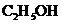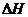Chapter 10, Problem 44QAP### Introductory Chemistry: A Foundati...

9th Edition
Steven S. Zumdahl + 1 other
ISBN: 9781337399425

#### Solutions

Chapter
Section### Introductory Chemistry: A Foundati...

9th Edition
Steven S. Zumdahl + 1 other
ISBN: 9781337399425
Textbook Problem
11 views

# When ethanol (grain alcohol,is burned in oxygen, approximately 1360 kJ of heat energy is released per mole of ethanol.mg src=Images/HTML_99425-10-44QAP_image002.jpg alt="" align="top"/> What quantity of heat is released for each gram of ethanol burned? i>What isfor the reaction as written? How much heat is released when sufficient ethanol is burned so as to produce 1 mole of water vapor?

Interpretation Introduction

(a)

Interpretation:

Calculate the heat produced when a specific amount of ethanol.

Concept Introduction:

Convert the number of moles for the provided mass of ethanol then calculate the heat produced per mole.

Explanation

Calculate heat produced for 1.00 g of ethanol as follows:

1.00gCH3CH2OH×1molCH3CH2OH46

Interpretation Introduction

(b)

Interpretation:

Understand what sign for exothermic reaction and endothermic reaction.

Concept Introduction:

In case the system absorbs heat, the process is endothermic reaction

Incase the system evolves heat, the process is exothermic reaction.

Interpretation Introduction

(c)

Interpretation:

Calculate the heat produced when a specific amount of water.

Concept Introduction:

Use the number of moles involved in the provide reaction then calculate heat released for 1 mole of water.

### Still sussing out bartleby?

Check out a sample textbook solution.

See a sample solution

#### The Solution to Your Study Problems

Bartleby provides explanations to thousands of textbook problems written by our experts, many with advanced degrees!

Get Started